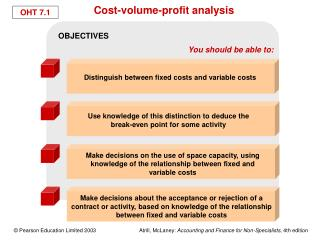# Cost-volume-profit analysis - PowerPoint PPT PresentationDownload PresentationCost-volume-profit analysis

Cost-volume-profit analysisDownload Presentation## Cost-volume-profit analysis

- - - - - - - - - - - - - - - - - - - - - - - - - - - E N D - - - - - - - - - - - - - - - - - - - - - - - - - - -
##### Presentation Transcript

1. OBJECTIVES You should be able to: Distinguish between fixed costs and variable costs Use knowledge of this distinction to deduce the break-even point for some activity Make decisions on the use of space capacity, using knowledge of the relationship between fixed and variable costs Make decisions about the acceptance or rejection of a contract or activity, based on knowledge of the relationship between fixed and variable costs Cost-volume-profit analysis

2. Costs may be broadly classified as Those that stay fixed (the same) when changes occur to the volume of activity Fixed Those that vary according to the volume of activity Variable The behaviour of costs

3. Cost (£) F 0 Volume of activity (units of output) Graph of fixed cost(s) against the volume of activity

4. Rent cost (£) R 0 Volume of activity Graph of rent cost against the volume of activity

5. Cost (£) 0 Volume of activity Graph of variable costs against the volume of activity

6. Graph of electricity cost against the volume of activity The slope of this line gives the variable cost per unit of activity Electricity cost (£) Fixed costelement 0 Volume of activity

7. Cost (£) Total cost Variable costs F Fixed costs 0 Volume of activity (units of output) Graph of total cost against the volume of activity

8. Total sales revenue Cost (£) Break-even point Total cost Variable costs F Fixed costs 0 Volume of activity (units of output) Break-even chart

9. Fixed costsSales revenue per unit – Variable costs per unit b = Calculating the break-even point

10. OHT 7.10a 5 Totalcosts Totalrevenue Cost (£000) 4 3 Break-evenpoint 2 1 Fixed costs 0 100 200 300 400 500 Volume of activity (number of baskets) Break-even chart for Cottage Industries’ basket-making without the machine

11. OHT 7.10b 6 Cost (£000) 5 Totalrevenue Totalcosts 4 3 Break-evenpoint 2 1 Fixed costs 0 100 200 300 400 500 Volume of activity (number of baskets) Break-even chart for Cottage Industries’ basket-making with the machine

12. OHT 7.12 The effect of operating gearing

13. OHT 7.12 Profit (£) Break-even point Profit 0 Volume of activity Fixed cost Loss Loss (£) Profit-volume chart

14. OHT 7.13 Three general problems Non-linear relationships Stepped fixed costs Multi-product businesses Weaknesses of break-even analysis

15. OHT 7.14 Can be used for the following short-term decisions: Accepting/rejecting special contracts The most effective use of scarce resources Make-or-buy decisions Closing or continuation decisions Marginal analysis